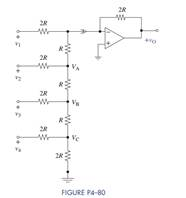Create an Account

Home / Questions / A fifth bit is added to the R-2R DAC shown in Figure P4–80. What is the maximum possible...

A fifth bit is added to the R-2R DAC shown in Figure P4–80. What is the maximum possible...

A fifth bit is added to the R-2R DAC shown in Figure P4–80. What is the maximum possible magnitude of the output voltage? What is the resolution of the revised DAC?Jul 28 2020 View more View LessSubscribe To Get Solution